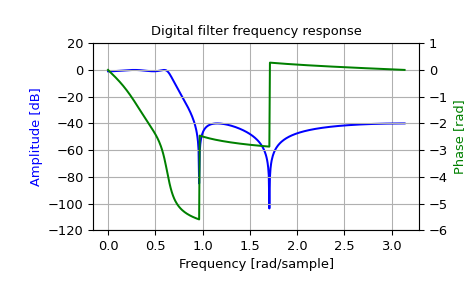# scipy.signal.iirdesign¶

scipy.signal.iirdesign(wp, ws, gpass, gstop, analog=False, ftype='ellip', output='ba', fs=None)[source]

Complete IIR digital and analog filter design.

Given passband and stopband frequencies and gains, construct an analog or digital IIR filter of minimum order for a given basic type. Return the output in numerator, denominator (‘ba’), pole-zero (‘zpk’) or second order sections (‘sos’) form.

Parameters
wp, wsfloat or array like, shape (2,)

Passband and stopband edge frequencies. Possible values are scalars (for lowpass and highpass filters) or ranges (for bandpass and bandstop filters). For digital filters, these are in the same units as fs. By default, fs is 2 half-cycles/sample, so these are normalized from 0 to 1, where 1 is the Nyquist frequency. For example:

• Lowpass: wp = 0.2, ws = 0.3

• Highpass: wp = 0.3, ws = 0.2

• Bandpass: wp = [0.2, 0.5], ws = [0.1, 0.6]

• Bandstop: wp = [0.1, 0.6], ws = [0.2, 0.5]

For analog filters, wp and ws are angular frequencies (e.g., rad/s). Note, that for bandpass and bandstop filters passband must lie strictly inside stopband or vice versa.

gpassfloat

The maximum loss in the passband (dB).

gstopfloat

The minimum attenuation in the stopband (dB).

analogbool, optional

When True, return an analog filter, otherwise a digital filter is returned.

ftypestr, optional

The type of IIR filter to design:

• Butterworth : ‘butter’

• Chebyshev I : ‘cheby1’

• Chebyshev II : ‘cheby2’

• Cauer/elliptic: ‘ellip’

• Bessel/Thomson: ‘bessel’

output{‘ba’, ‘zpk’, ‘sos’}, optional

Filter form of the output:

• second-order sections (recommended): ‘sos’

• numerator/denominator (default) : ‘ba’

• pole-zero : ‘zpk’

In general the second-order sections (‘sos’) form is recommended because inferring the coefficients for the numerator/denominator form (‘ba’) suffers from numerical instabilities. For reasons of backward compatibility the default form is the numerator/denominator form (‘ba’), where the ‘b’ and the ‘a’ in ‘ba’ refer to the commonly used names of the coefficients used.

Note: Using the second-order sections form (‘sos’) is sometimes associated with additional computational costs: for data-intense use cases it is therefore recommended to also investigate the numerator/denominator form (‘ba’).

fsfloat, optional

The sampling frequency of the digital system.

New in version 1.2.0.

Returns
b, andarray, ndarray

Numerator (b) and denominator (a) polynomials of the IIR filter. Only returned if `output='ba'`.

z, p, kndarray, ndarray, float

Zeros, poles, and system gain of the IIR filter transfer function. Only returned if `output='zpk'`.

sosndarray

Second-order sections representation of the IIR filter. Only returned if `output=='sos'`.

`butter`

Filter design using order and critical points

`cheby1`, `cheby2`, `ellip`, `bessel`
`buttord`

Find order and critical points from passband and stopband spec

`cheb1ord`, `cheb2ord`, `ellipord`
`iirfilter`

General filter design using order and critical frequencies

Notes

The `'sos'` output parameter was added in 0.16.0.

Examples

```>>> from scipy import signal
>>> import matplotlib.pyplot as plt
>>> import matplotlib.ticker
```
```>>> wp = 0.2
>>> ws = 0.3
>>> gpass = 1
>>> gstop = 40
```
```>>> system = signal.iirdesign(wp, ws, gpass, gstop)
>>> w, h = signal.freqz(*system)
```
```>>> fig, ax1 = plt.subplots()
>>> ax1.set_title('Digital filter frequency response')
>>> ax1.plot(w, 20 * np.log10(abs(h)), 'b')
>>> ax1.set_ylabel('Amplitude [dB]', color='b')
>>> ax1.grid()
>>> ax1.set_ylim([-120, 20])
>>> ax2 = ax1.twinx()
>>> angles = np.unwrap(np.angle(h))
>>> ax2.plot(w, angles, 'g')